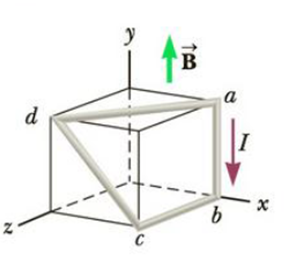Chapter 19, Problem 33P

Chapter
Section
Textbook Problem

In Figure P19.33, the cube is 40.0 cm on each edge. Four straight segments of wire—ab, bc, cd, and da—form a closed loop that carries a current I = 5.00 A in the direction shown. A uniform magnetic field of magnitude B = 0.020 0 T is in the positive y-direction. Determine the magnitude and direction of the magnetic force on each segment.Figure P19.33

(a)

To determine
The direction and the magnitude of the magnetic force on the wire segment ab.

Explanation

Given info: The length of the edge of the cube is 40.0cm . The current through the segments of wire is 5.00A . The uniform magnetic field is 0.020T acting in the positive y-direction.

Explanation:

The magnitude of the magnetic force on a current carrying wire is given by,

Fm=BIlsinθ

• B

(b)

To determine
The direction and the magnitude of the magnetic force on the wire segment bc.

(c)

To determine
The direction and the magnitude of the magnetic force on the wire segment cd.

(d)

To determine
The direction and the magnitude of the magnetic force on the wire segment da.

Still sussing out bartleby?

Check out a sample textbook solution.

See a sample solution

The Solution to Your Study Problems

Bartleby provides explanations to thousands of textbook problems written by our experts, many with advanced degrees!

Get Started